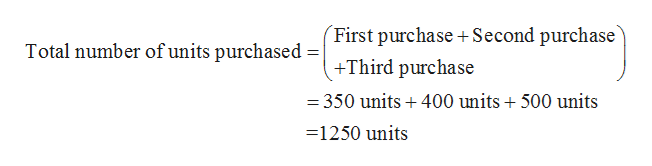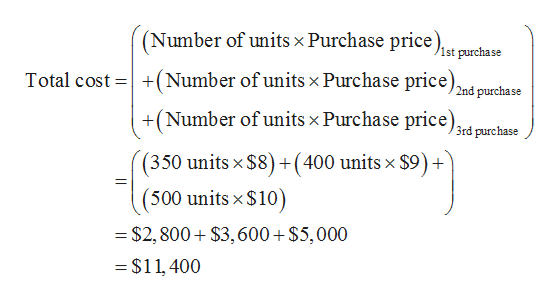# In its first month of operations, Sheffield Corp. made three purchases of merchandise in the following sequence: (1) 350 units at \$8, (2) 400 units at \$9, and (3) 500 units at \$10. Calculate average unit cost. (Round answer to 3 decimal places, e.g. 5.125.)Average unit cost \$___________________

Question
11 views

In its first month of operations, Sheffield Corp. made three purchases of merchandise in the following sequence: (1) 350 units at \$8, (2) 400 units at \$9, and (3) 500 units at \$10.

Calculate average unit cost. (Round answer to 3 decimal places, e.g. 5.125.)

 Average unit cost \$___________________

check_circle

Step 1

Hence, the total number of units purchased is 1250 units. It is the sum of first, second and third purchase.help_outlineImage Transcriptionclose(First purchase Second purchase Total number of units purchased +Third purchase = 350 units+400 units + 500 units 1250 units fullscreen
Step 2

Hence, \$11,400 is the total cost. It is obtained by the addition of the product...help_outlineImage Transcriptionclose(Number of units x Purchase price. 1st purchase Total cost (Number of units x Purchase price) 2nd purchase +(Number of units x Purchase price) 3rd purchase (350 units x \$8) +(400 units x \$9)+ (500 units x \$10) \$2,800 \$3,600 \$5,000 =\$11 400 fullscreen

### Want to see the full answer?

See Solution

#### Want to see this answer and more?

Solutions are written by subject experts who are available 24/7. Questions are typically answered within 1 hour.*

See Solution
*Response times may vary by subject and question.
Tagged in